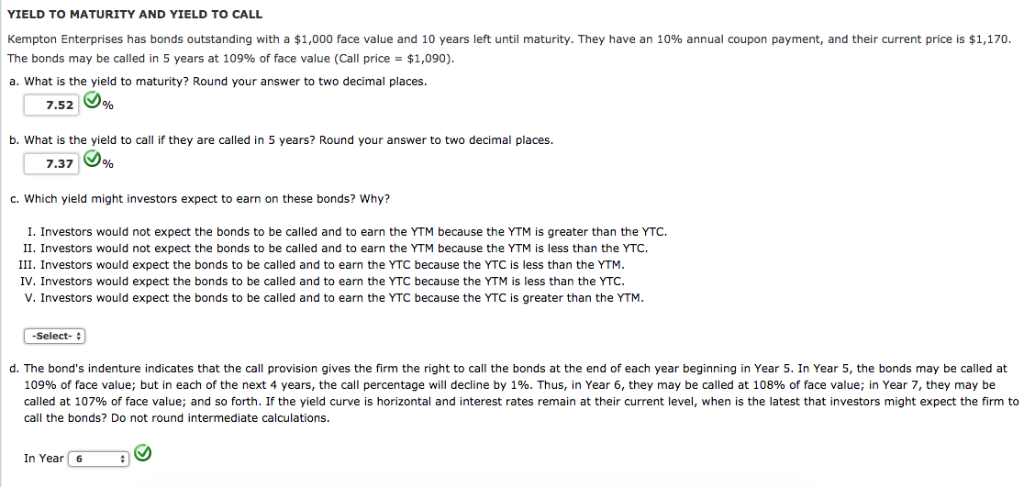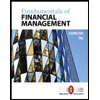# YIELD TO MATURITY AND YIELD TO CALL Kempton Enterprises has bonds outstanding with a \$1,000 face value and 10 years left until maturity. They have an 10% annual coupon payment, and their current price is \$1,170. The bonds may be called in 5 years at 109% of face value (Call price \$1,090). a. What is the yield to maturity? Round your answer to two decimal places. 7.52 b. What is the yield to call if they are called in 5 years? Round your answer to two decimal places. 7.37 earn on these bonds? Why? c. Which yield might investors expect be called and to earn the YTM because the YTM is greater than the YTC I. Investors would not expect the bonds II. Investors would not expect the bonds be called and to earn the YTM because the YTM is less than the YTC. III. Investors would expect the bonds to be called and to earn the YTC because the YTC is less than the YTM. IV. Investors would expect the bonds to be called and to earn the YTC because the YTM is less than the YTC V. Investors would expect the bonds to be called and to earn the YTC because the YTC is greater than the YTM. -Select- d. The bond&#39;s indenture indicates that the call provision gives the firm the right to calll the bonds at the end of each year beginning in Year 5. In Year 5, the bonds may be called at a te 109% of face value; but in each of the next 4 years, the call percentage will decline by 1%. Thus, in Year 6, they may be called at 108% of face value; in Year 7, they may be called at 107 % of face value; and so forth. If the yield curve is horizontal and interest rates remain at their current level, when is the latest that investors might expect the firm to call the bonds? Do not round intermediate calculations. In Year 6

QuestionYIELD TO MATURITY AND YIELD TO CALL Kempton Enterprises has bonds outstanding with a \$1,000 face value and 10 years left until maturity. They have an 10% annual coupon payment, and their current price is \$1,170. The bonds may be called in 5 years at 109% of face value (Call price \$1,090). a. What is the yield to maturity? Round your answer to two decimal places. 7.52 b. What is the yield to call if they are called in 5 years? Round your answer to two decimal places. 7.37 earn on these bonds? Why? c. Which yield might investors expect be called and to earn the YTM because the YTM is greater than the YTC I. Investors would not expect the bonds II. Investors would not expect the bonds be called and to earn the YTM because the YTM is less than the YTC. III. Investors would expect the bonds to be called and to earn the YTC because the YTC is less than the YTM. IV. Investors would expect the bonds to be called and to earn the YTC because the YTM is less than the YTC V. Investors would expect the bonds to be called and to earn the YTC because the YTC is greater than the YTM. -Select- d. The bond&#39;s indenture indicates that the call provision gives the firm the right to calll the bonds at the end of each year beginning in Year 5. In Year 5, the bonds may be called at a te 109% of face value; but in each of the next 4 years, the call percentage will decline by 1%. Thus, in Year 6, they may be called at 108% of face value; in Year 7, they may be called at 107 % of face value; and so forth. If the yield curve is horizontal and interest rates remain at their current level, when is the latest that investors might expect the firm to call the bonds? Do not round intermediate calculations. In Year 6
Expert Solution

### Want to see the full answer?

Check out a sample Q&A hereStudents who’ve seen this question also like:Fundamentals of Financial Management, Concise Edition (MindTap Course List)
9th Edition
ISBN: 9781305635937
Author: Eugene F. Brigham, Joel F. Houston
Publisher: Cengage Learning